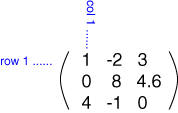# Write a C++ Program to Print Lowerhalf and Upperhalf of Triangle Matrix

By | 27.12.2016

## C++ Program to Print Lowerhalf and Upperhalf  of  Triangle Matrix

Write a C++ Program to Print Lowerhalf and Upperhalf of Triangle Matrix. Here’s a Simple Program to Print Lowerhalf and Upperhalf of Triangle Matrix in C++ Programming Language.

## Definition of Matrix

• A matrix is a collection of numbers arranged into a fixed number of rows and columns. Usually the numbers are real numbers. In general, matrices can contain complex numbers but we won’t see those here.
• Here is an example of a matrix with three rows and three columns:

##• The top row is row 1. The leftmost column is column 1. This matrix is a 3×3 matrix because it has three rows and three columns.
• In describing matrices, the format is:

rows X columns

• Each number that makes up a matrix is called an element of the matrix. The elements in a matrix have specific locations.
• The upper left corner of the matrix is row 1 column 1. In the above matrix the element at row 1 col 1 is the value 1. The element at row 2 column 3 is the value 4.6.

Below is the source code for C++ Program to Print Lowerhalf and Upperhalf of Triangle Matrix which is successfully compiled and run on Windows System to produce desired output as shown below :

### SOURCE CODE : :

```// C++ Program to Print Lowerhalf and Upperhalf of Triangle Matrix

#include<iostream>

using namespace std;

int main()
{
int a,i,j,m;
cout<<"Enter size of the Matrix(min:3,max:5):";
cin>>m;
cout<<"\nEnter the Matrix row wise:\n";

for(i=0;i<m;i++)
for(j=0;j<m;++j)
cin>>a[i][j];

cout<<"\n\n";

cout<<"Upperhalf  of  Triangle Matrix :: \n";

for(i=0;i<m;++i)
{
for(j=0;j<m;++j)
{
if(i<j)
cout<<a[i][j]<<" ";
else
cout<<"  ";
}

cout<<"\n";
}

cout<<"\n";

cout<<"Lowerhalf  of  Triangle Matrix :: \n";

for(i=0;i<m;++i)
{
for(j=0;j<m;++j)
{
if(j<i)
cout<<a[i][j]<<" ";
else
cout<<" ";
}
cout<<"\n";
}

return 0;
}```

### OUTPUT : :

```*******************OUTPUT*********************

*******************FIRST RUN******************

Enter size of the Matrix(min:3,max:5):3

Enter the Matrix row wise:
1
2
3
4
5
6
7
8
9

Upperhalf  of  Triangle Matrix ::
2 3
6

Lowerhalf  of  Triangle Matrix ::

4
7 8

*******************SECOND RUN******************

Enter size of the Matrix(min:3,max:5):4

Enter the Matrix row wise:
3
1
2
4
6
5
9
7
2
6
7
9
1
3
2
0

Upperhalf  of  Triangle Matrix ::
1 2 4
9 7
9

Lowerhalf  of  Triangle Matrix ::

6
2 6
1 3 2```

If you found any error or any queries related to the above program or any questions or reviews , you wanna to ask from us ,you may Contact Us through our contact Page or you can also comment below in the comment section.We will try our best to reach upto you in the short interval.

Article Rating
Category: Arrays Programs C++ Programming Tags: ,